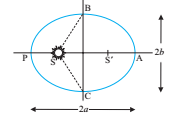The three laws of Kepler can be stated as follows:

### Kepler’s first law (law of orbit):

Every planet revolves around the sun in an elliptical orbit with the sun is situated at one focus of the ellipse.An ellipse traced out by a planet around the sun. The closest point is P and the farthest point is A, P is called the perihelion and A the aphelion. The semi major axis is half the distance AP.

### Kepler’s second law (law of area):

The radius vector drawn from the sun to a planet sweeps out equal areas in equal intervals of time, i.e., the areal velocity of the planet around the sun is constant.The planet P moves around the sun in an elliptical orbit. The shaded area is the area ΔA swept out in a small interval of time Δt.

### Kepler’s third law (law of period):

The square of the time period of revolution of a planet around the sun is directly proportional to the cube of semi major axis of the elliptical orbit of the planet around the sun.

Click on a star to rate it!

Average rating 0 / 5. Vote count: 0

No votes so far! Be the first to rate this post.

As you found this post useful...